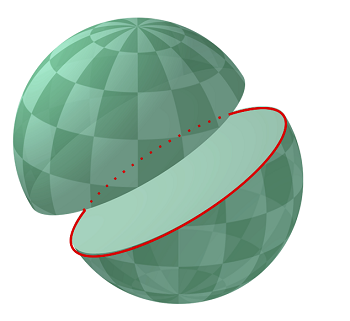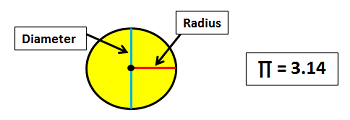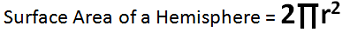# Surface Area of a Hemisphere

Instructor: Nola Bridgens

Nola has taught elementary school and tutored for four years. She has a bachelor's degree in Elementary Education, a master's degree in Marketing, and is a certified teacher.

In this lesson, we will discuss the elements of a hemisphere and define surface area. We will also learn the formula to calculate the surface area of a hemisphere and how to apply it.

## Hemispheres and Surface Area

Have you ever heard the terms, 'Northern and Southern Hemispheres', when referring to the Earth? A hemisphere is precisely half of a sphere, which is a perfectly round 3-dimensional object, such as a ball. Imagine cutting one of your own balls straight through the middle, which would give you a hemisphere, as shown in this image.Surface area is the sum of all the sides of a 3-dimensional object. In the case of a sphere, think about an orange. If you were to peel an orange, its surface area would be the total amount of the peel. The surface area of a hemisphere would be like the peel of half of the orange.

## Elements of a Hemisphere

Before we learn the formula for the surface area of a hemisphere, we need to review the elements of a hemisphere:

• The radius is a line that connects the center point of a circle or sphere with the outside layer, or circumference.
• The diameter is twice the radius or distance from one side of the circumference to the other through the center point.
• TT is the symbol we use for pie and it represents the ratio of the circumference of a circle to its diameter. TT always equals 3.14.## Formula and Examples

As long as you know the radius of a hemisphere, the formula we use to calculate its surface area is very straightforward.Let's try an example: find the surface area of a hemisphere with a radius of 5 inches.

2TTr2 = (2)(3.14)(52) = (6.28)(52) = (6.28)(25) = 157 square inches.

A hemisphere with a radius of 5 inches has a surface area of 157 square inches.

But what if we only know the diameter of the hemisphere? Well, we know that the diameter is equal to two times the radius. So, we can divide the diameter by 2 to find the radius.

Let's try another example: find the surface area of a hemisphere with a diameter of 8 feet.

To unlock this lesson you must be a Study.com Member.

### Register to view this lesson

Are you a student or a teacher?

#### See for yourself why 30 million people use Study.com

##### Become a Study.com member and start learning now.
Back
What teachers are saying about Study.com

### Earning College Credit

Did you know… We have over 200 college courses that prepare you to earn credit by exam that is accepted by over 1,500 colleges and universities. You can test out of the first two years of college and save thousands off your degree. Anyone can earn credit-by-exam regardless of age or education level.